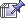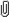September 26, 2023, 01:22:16 pm

###AuthorTopic: Mathematics Question Thread  (Read 1234701 times) Tweet Share

0 Members and 5 Guests are viewing this topic.

#### Maroon and Gold Never Fold

• Trailblazer
•• Posts: 33
• Respect: 0« Reply #4545 on: July 20, 2021, 09:02:54 pm »
0
For this inequality
I initially attempted it by making it into this like I would with any absolute function
This did not work, I looked at the solutions and they revomed the absolute value bars, why is this? is anyone able to explain to me how to do this inequality.

Thanks

#### fun_jirachi

• MOTM: AUG 18
• Moderator
• Part of the furniture
••• Posts: 1068
• All doom and Gloom.
• Respect: +710« Reply #4546 on: July 20, 2021, 09:27:45 pm »
+2
It might be more helpful if you post your working; personally I'd like to see exactly where you went wrong. It's risky to play around inequalities and absolute values (in that you can easily get the question wrong). Graphing (using general sketches) is a lot more low risk in general.

As for why they removed the absolute value bars, it's because they can; you can always construct a solution that is logically/mathematically identical but without them.

Please post your working, I'd really like to see it(if required I'll post an actual solution afterwards)
« Last Edit: July 21, 2021, 10:37:28 am by fun_jirachi »
Spoiler
HSC 2018: Mod Hist  | 2U Maths 
HSC 2019: Physics  | Chemistry  | English Adv  | 3U Maths  | 4U Maths 
ATAR: 99.05

UCAT: 3310 - VR  | DM  | QR  | AR 
Subject Acceleration (2018)
UCAT Question Compilation/FAQ (2020)

#### 1729

• MOTM: July 20
• Trendsetter
•• Posts: 185
• Respect: +158« Reply #4547 on: July 20, 2021, 10:09:14 pm »
+3
For this inequality
I initially attempted it by making it into this like I would with any absolute function
This did not work, I looked at the solutions and they revomed the absolute value bars, why is this? is anyone able to explain to me how to do this inequality.

Thanks

If we have function $f(x)$, and make it $\left|f\left(x\right)\right|$ it essentially flips any cordinates of $f\left(x\right)<0$ (cordinates that lie under the $y$ axis) over the $x$ axis since absolute values make negative numbers positive by definition, ie. $\left|-p\right|=p$ and $\left|p\right|=p$

Notice how in the function you sent there is nothing to flip over the $x$ axis or there isn't anything below $y=0$? In this case, your function is equal to the absolute value of it since it's always positive similarly, if p is positive, the absolute value of p is just itself $\left|p\right|=p$ ie. $\frac{x^2}{\left(1-x\right)^2}>\:0$ is always more than 0 (positive)

« Last Edit: July 20, 2021, 10:23:45 pm by 1729 »

#### Maroon and Gold Never Fold

• Trailblazer
•• Posts: 33
• Respect: 0« Reply #4548 on: July 21, 2021, 10:23:06 am »
0

If we have function $f(x)$, and make it $\left|f\left(x\right)\right|$ it essentially flips any cordinates of $f\left(x\right)<0$ (cordinates that lie under the $y$ axis) over the $x$ axis since absolute values make negative numbers positive by definition, ie. $\left|-p\right|=p$ and $\left|p\right|=p$

Notice how in the function you sent there is nothing to flip over the $x$ axis or there isn't anything below $y=0$? In this case, your function is equal to the absolute value of it since it's always positive similarly, if p is positive, the absolute value of p is just itself $\left|p\right|=p$ ie. $\frac{x^2}{\left(1-x\right)^2}>\:0$ is always more than 0 (positive)

So because the function is positive for all values of x, we can remove the absolute value bars because they make no difference?

#### 1729

• MOTM: July 20
• Trendsetter
•• Posts: 185
• Respect: +158« Reply #4549 on: July 21, 2021, 10:28:12 am »
+3
So because the function is positive for all values of x, we can remove the absolute value bars because they make no difference?
Yep that is correct.

Think about it, if you take the absolute value of a positive number, you are left with the same number! This rule applies to functions as well (:

#### crippledbyenglish

•• Posts: 10
• Respect: 0« Reply #4550 on: November 11, 2021, 05:24:23 pm »
0

____________________________
(Image removed from quote.)

I want to let you have a go at (iii) first. Use a graph similar to the graph in (ii). Come back with whatever progress you have.

#### crippledbyenglish

•• Posts: 10
• Respect: 0« Reply #4551 on: November 12, 2021, 10:40:09 am »
0
Hi everyone! Just wondering if someone could let me know how they would have thought about the question below, needing to find tan from sin. I understand how they got the answer but didn't think to simplify the denominator by factorising. What would you look for/what hints would you take from the question to do this?

Thanks!

#### crippledbyenglish

•• Posts: 10
• Respect: 0« Reply #4552 on: November 12, 2021, 10:45:16 am »
0
When I looked at the solutions for the question below it said that you should dilate and then translate which is weird because aren't you supposed to do what is in the parenthesis first? Is this a misconception?

#### crippledbyenglish

•• Posts: 10
• Respect: 0« Reply #4553 on: November 12, 2021, 10:51:54 am »
0
Someone please help I don't think I know how to use a calculator#### SnekiSnek« Reply #4554 on: November 12, 2021, 02:10:13 pm »
0
When I looked at the solutions for the question below it said that you should dilate and then translate which is weird because aren't you supposed to do what is in the parenthesis first? Is this a misconception?

I am in VCE not HSC but for methods it was to follow DRT
Dilations first, then reflections and then translations
Literature, Methods, Further, Chemistry, Legal Studies, Psychology, Classical Studies, Physics

#### fun_jirachi

• MOTM: AUG 18
• Moderator
• Part of the furniture
••• Posts: 1068
• All doom and Gloom.
• Respect: +710« Reply #4555 on: November 12, 2021, 03:14:58 pm »
+1

Have you had a go at the last part? That's the advice given in the original response, and I'm reluctant to deviate from it because I think it's 100% correct - you should have a go first.

Hi everyone! Just wondering if someone could let me know how they would have thought about the question below, needing to find tan from sin. I understand how they got the answer but didn't think to simplify the denominator by factorising. What would you look for/what hints would you take from the question to do this?

Thanks!

This sort of factorisation should be relatively standard as you do look into completing the square when you study solutions to quadratics. It's in a different form so it may not be immediately obvious, but you should be on the lookout for things like this regardless. More of a practice thing to get your eye in on these sorts of tricks. It's not strictly necessary to employ this trick in this particular instance, in any case.

When I looked at the solutions for the question below it said that you should dilate and then translate which is weird because aren't you supposed to do what is in the parenthesis first? Is this a misconception?

You're thinking of computing equations. SnekiSnek is correct

Someone please help I don't think I know how to use a calculator$z = \frac{x - \mu}{\sigma}$. If the numbers in the table were all correct, $\mu, \sigma$ would be equal in a system of linear equations. Solve two simultaneously to find values of $\mu, \sigma$ then sub into the other result to see what adjustment needs to be made (and if it matches one of the other options given).

I'm not giving you the answer because I don't really see an attempt ('someone please help' is not really helpful to me because I want to help you target the bits you're struggling with, I'm only able to give you general advice in this case).

In future, please add more working and refrain from doing a double post, let alone a quadruple
Spoiler
HSC 2018: Mod Hist  | 2U Maths 
HSC 2019: Physics  | Chemistry  | English Adv  | 3U Maths  | 4U Maths 
ATAR: 99.05

UCAT: 3310 - VR  | DM  | QR  | AR 
Subject Acceleration (2018)
UCAT Question Compilation/FAQ (2020)

#### Albertenouttaten

• Trailblazer
•• Posts: 25
• Respect: 0« Reply #4556 on: March 10, 2022, 06:03:32 pm »
0
Normal Distribution question that is absolutely melting my mind. Even the wording of the question is so tuff. Any tips and guidance on how to do this would be greatly appreciated.

#### 1729

• MOTM: July 20
• Trendsetter
•• Posts: 185
• Respect: +158« Reply #4557 on: March 11, 2022, 01:24:12 pm »
+1
Normal Distribution question that is absolutely melting my mind. Even the wording of the question is so tuff. Any tips and guidance on how to do this would be greatly appreciated.

For the purpose of my explanation let $X$ be the normally distributed random variable such that $X$~$N\left(\mu ,3^2\right)$. We want to find out what $\mu$ is given that $\text{Pr}\left(X\le 29\right)=0.16$.

I want you to consider that $\text{Pr}\left(X<a\right)=\text{Pr}\left(Z<\frac{a-\mu }{\sigma }\right)$ where $Z$ is the standard normal random variable.

Now solve $\text{Pr}\left(Z\le \frac{29-\mu }{6}\right)=0.16$ for $\mu$.

However, this method assumes access to technology to get a more accurate approximation.

If you did not have access to CAS, you could simply use the fact that approximately $68$% of values lie within 1 standard deviation of the mean, that is $\mu -\sigma <x<\mu +\sigma$. This means that $32$% lie everywhere else, which means that $16$% lie $x<\mu -\sigma$.

This essentially means that $\text{Pr}\left(X<\mu -\sigma \right)\approx \text{Pr}\left(X<29\right)\approx 0.16$. Now solve $29=\mu -\sigma$ or $29=\mu -6$.
« Last Edit: March 11, 2022, 02:20:57 pm by 1729 »

#### Sagarc

• Fresh Poster
•• Posts: 2
• Respect: 0« Reply #4558 on: May 30, 2022, 11:31:42 pm »
0
I am stuck on this question from the book. Any help appreciated.

A flat verandah roof 1.8 m deep is 2.6 m up from the ground. At a certain time of day, the sun makes an angle of elevation of 72°25′. How much shade is provided on the ground by the verandah roof at that time, to 1 decimal place?

#### fun_jirachi

• MOTM: AUG 18
• Moderator
• Part of the furniture
••• Posts: 1068
• All doom and Gloom.
• Respect: +710« Reply #4559 on: June 01, 2022, 10:55:42 am »
0
This seems a little vague - is there a particular book you can reference or a diagram you can display? I can't quite infer what the intended meaning of the question is
Spoiler
HSC 2018: Mod Hist  | 2U Maths 
HSC 2019: Physics  | Chemistry  | English Adv  | 3U Maths  | 4U Maths 
ATAR: 99.05

UCAT: 3310 - VR  | DM  | QR  | AR